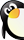### 一年级上数学单元测试一年级数学上册123测试题卷人教新课标.• 0个馈赠点
• 0个贡献点
• 0个黄金点

• 资源类别 试题
资源子类 单元测试
• 教材版本 人教版
所属学科 小学数学
• 适用年级 一年级
适用地区 全国通用
• 文件大小 1043 K
上传用户 goldfisher
• 更新时间 2018/10/16 18:33:33
下载统计 今日0 总计47
• 评论(0) 发表评论 报错(0) 我要报错 收藏
3
2

 2 + 3= 2－2= 4－2= 2 + 0= 4－3= 3 + 2= 2 +2= 5－2= 0 + 3= 5－3= 2－0= 3－2= 5－1= 3 + 1= 2－1= 2 + 1= 1 + 1= 1 + 3= 1 + 4= 1－0=

• 暂时没有相关评论